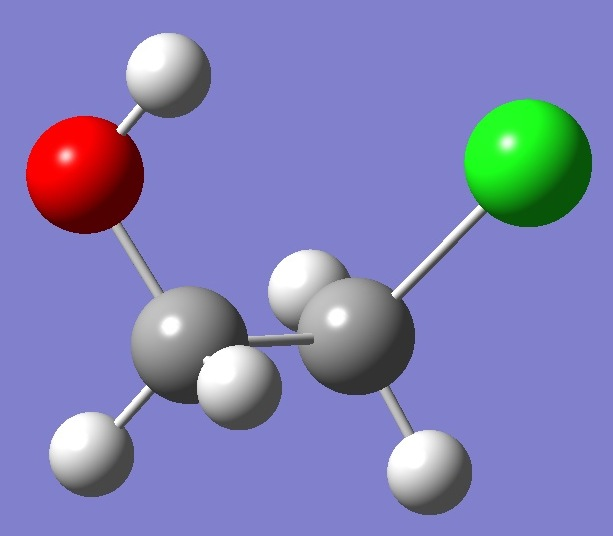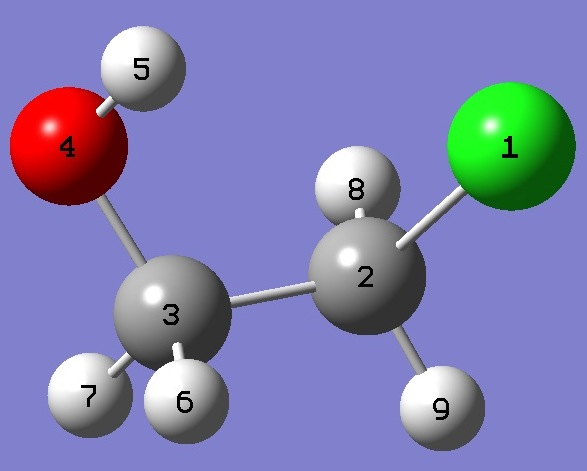ClH2C-CH2OHChlorine

in 2-Chloroethanol

Calculation of the chlorine nqcc tensors in 2-chloroethanol was made on molecular structures given by MP2/6-311+G(3df,3pd) optimization with empirically corrected approximate equilibrium C-Cl and C-C bond lengths, and by MP2/aug-cc-pVTZ optimization also with empirically corrected approximate equilibrium C-Cl and C-C bond lengths.  Calculated nqcc's are compared with experimental values  in Tables 1 and 2.  Structure parameters are given in Z-Matrix format in Table 3, rotational constants and dipole moments in Table 4.

In Tables 1 and 2, subscripts a,b,c refer to the principal axes of the inertia tensor; x,y,z to the principal axes of the nqcc tensor.  ETA = (Xxx - Xyy)/Xzz.  Øz,CCl (degrees) is the angle the z-principal axis makes with the CCl bond axis.

RMS is the root mean square difference between calculated and experimental diagonal nqcc's (percentage of the average of the magnitudes of the experimental nqcc's).  RSD is the calibration residual standard deviation of the B1LYP/TZV(3df,2p) model for calculation of the chlorine efg's/nqcc's.

 Table 1.  35Cl nqcc's in 2-Chloroethanol (MHz).  Calculation was made on structures given by (1) MP2/6-311+G(3df,3pd) and (2) MP2/aug-cc-pVTZ optimization, each with approximate re C-Cl and C-C bond lengths. Calc. (1) Calc. (2) Expt.  Xaa - 19.27 - 18.77 - 19.16(10) Xbb - 12.77 - 13.32 - 12.65(10) Xcc 32.05 32.09 31.82(10) Xab * - 49.25 - 49.29 50(2) Xac * 14.88 14.77 14.7(3) Xbc * 15.41 15.44 - 14.0(10) RMS 0.16 (0.78 %) 0.47 (2.2 %) RSD 0.49 (1.1 %) 0.49 (1.1 %) Xxx 33.01 32.93 Xyy 36.86 36.86 Xzz - 69.87 - 69.79 - 70.0(20) ETA 0.0550 0.0562 0.02(3) Øz,CCl 0.85 0.86

* The algebraic signs of the off-diagonal components depend on the orientation of the molecule with respect to a,b,c axes.  In this regard, calculated and experimental off-diagonal nqcc's can be brought into agreement by transformation: b --> -b or a --> -a and c --> -c.

 Table 2.  37Cl nqcc's in 2-Chloroethanol (MHz).  Calculation was made on structures given by (1) MP2/6-311+G(3df,3pd) and (2) MP2/aug-cc-pVTZ optimization, each with approximate re C-Cl and C-C bond lengths. Calc. (1) Calc. (2) Expt. Xaa - 15.70 - 15.30 - 15.84(10) Xbb - 9.61 - 10.03 - 9.42(10) Xcc 25.31 25.33 25.27(10) Xab - 38.80 - 38.77 Xac 11.72 11.64 Xbc 12.00 12.02 RMS 0.14 (0.83 %) 0.47 (2.8 %) RSD 0.44 (1.1 %) 0.44 (1.1 %)

 Table 3.  2-Chloroethanol.  Structure parameters (Å and degrees).  Approximate re bond lenths are given in parentheses.Cl  C,1,R1  C,2,R2,1,A1  O,3,R3,2,A2,1,D1,0  H,4,R4,3,A3,2,D2,0  H,3,R5,2,A4,1,D3,0  H,3,R6,2,A5,1,D4,0  H,2,R7,1,A6,3,D5,0  H,2,R8,1,A7,3,D6,0 MP2/6-311+G(3df,3pd) MP2/aug-cc-pVTZ R1=1.78219738   (1.7867)  R2=1.51210362   (1.5104)  R3=1.41022216  R4=0.96215182  R5=1.09291457  R6=1.08929165  R7=1.08598167  R8=1.0867186  A1=110.09482652  A2=112.43595836  A3=107.26954771  A4=109.48916893  A5=108.4236985  A6=107.06276072  A7=107.31747122  D1=63.95663716  D2=-59.63409202  D3=-60.58830708  D4=-178.78939566  D5=120.56993904  D6=-121.08036576 R1=1.7921435     (1.7889)  R2=1.51141363   (1.5098)  R3=1.41458903  R4=0.96539367  R5=1.09353056  R6=1.0902478  R7=1.08638571  R8=1.08716099  A1=109.76377351  A2=112.36352182  A3=106.99111482  A4=109.59066802  A5=108.53672498  A6=106.72701824  A7=107.09039208  D1=63.40574466  D2=-58.64933989  D3=-61.05150382  D4=-179.37280851  D5=120.55644685  D6=-121.16051388

 Table 4. 2-Chloroethanol-35Cl.  Rotational constants (MHz).  Calc(1) = MP2/6-311+G(3df,3pd) and Calc(2) = MP2/aug-cc-pVTZ optimization, each with approximate re C-Cl and C-C bond lengths. Calc (1) Calc (2) Expt.  A 12 866.4 12 759.8 12 747.976(30) B 3 538.9 3 560.4 3 505.777(20) C 3 011.2 3 021.1 2 981.276(20) |µa| 0.07 0.10 |µb| 1.48 1.53 |µc| 0.34 0.32

 R.G.Azrak and E.B.Wilson, J.Chem.Phys. 52,5299(1970).

CH3Cl CH3CH2Cl CH2ClCHF2 CH3CCl3

CF2ClCH3 CF2ClCHF2 CF2ClCH2F CF2ClCF3

CF3Cl CH2ClCF3 CH2ClCH2F CH3CHFCl

Molecules/Chlorine

ClH2CCH2OH.html